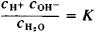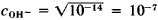# pH Value

Also found in: Dictionary, Thesaurus, Medical, Financial, Wikipedia.
The following article is from The Great Soviet Encyclopedia (1979). It might be outdated or ideologically biased.

## pH Value

a negative decimal logarithm of the hydrogen ion concentration, or more accurately, hydrogen ion activity (in gram ion per liter), of a given solution: pH = -logcH+. The pH value serves as a quantitative measure of a solution’s acidity, which has a significant influence on the direction and speed of many chemical and biochemical processes. At normal temperatures (more accurately, at 22° C) pH = 7 for neutral solutions, pH < 7 for acidic solutions, and pH > 7 for alkaline solutions. The accurate measurement and regulation of pH are necessary not only for chemical and biochemical laboratory research but also for numerous technological processes in industry, for the evaluation of soil properties, and for initiating procedures to increase the soil’s productivity.

Water dissociates into ions as shown by the equation H2O ⇄ H+ + OH-. According to the law of mass action,where c is the molar concentration and K is a constant at a given temperature (1.8 × 10-16 gram ion per liter at 22° C). One liter of water contains 1,000/18.016 = 55.56 gram moles of water (the quantity of dissociated molecules is so small that it can be ignored). Therefore, Kω = CH+ × COH- = K × cH2o = 1.8 × 10-16 × 55.56 = 1 x 10-14 (at 22° C). The constant Kωw is called the ion product of water. Because CH+ = COH- =in pure water (and in any neutral medium), pH = -log CH+ = 7. When acid is added to water, the CH+ increases and the pH decreases accordingly. So, for 0.01 molar (M) solution of HCl, the concentration CH+ = 10-2 and pH = -log CH+ = 2. When alkali is added to water, the COH- increases; then, CH+ = 10-14/cOH- decreases and the pH increases accordingly. For example, in a 0.01 M solution NaOH COH- = 10-2. This means that cH+ = 10-14/cOH- = 10-12 and pH = 12.

Poteiitiometric methods are primarily used for accurate pH measurements. When the determination of pH does not require high accuracy, pH is measured using a group of indicators, with each indicator changing its color at a certain pH. The pH scale is usually used for solutions that have a hydrogen ion concentration of 1 gram ion per liter and less. A neutral water solution has a pH of 7 only at room temperature. At higher temperatures dissociation of water increases, Kω increases, and at 100° C pure water has a pH of approximately 6. At temperatures below 22° C, pure water has a pH greater than 7. However, in all cases CH+ = COH- in a neutral medium.

### REFERENCE

Kireev, V. A. Kratkii kurs fizicheskoi khimii, 4th ed. Moscow, 1969.

V. L. VASILEVSKII

## pH value

A number denoting the degree of acidity or of basicity (alkalinity); 7 is a neutral value; acidity increases with decreasing values below 7; basicity increases with increasing values above 7.
McGraw-Hill Dictionary of Architecture and Construction. Copyright © 2003 by McGraw-Hill Companies, Inc.
References in periodicals archive ?
The optimum temperature is 37 AdegC and the pH value is 7.5 for maximum biogas production from the mixture of kitchen waste with inoculum.
The initial pH value of the fermentation media has an important bearing on the production of cephalosporin.
In the early stage of experiment, the soaking solution was titrated with sulfuric acid when the measured pH value was up to the titration pH value; however, in the final stage of experiment, even if the measured pH value was not up to the titration pH value, the soaking solution was still titrated with sulfuric acid to the initial pH value.
It has been reported that the increase in pH value was due to the process of deamination of amino acids resulting in the production of N[H.sub.3] and the metabolism of lactic acid bacteria to produce C[O.sub.2] .
The green sunlight laundry soap was the most (70%) frequently used soap and it showed one of the highest pH values of 10.34.
The pH value of the conventional and nanofluids were measured using pH meter (Fig.7) and reported in the table.6.
Bombay bakery biscuits (6.87) and Panna Cassata (5.95) biscuits found similarly in pH values and appeared more (P 0.05) from the biscuits of other bakeries examined in the present study.
The eight yeasts selected (34, 67, 80, 97, 205, 222, 225, 283) had different production of 1-kestose (p < 0.05) at the pH value of 4.5, at the temperatures 40, 50 and 60[degrees]C and, also, at pH values 5.5 and 6.5, at the temperatures 40 and 60[degrees]C.
Figure 4 shows that the aspect ratio in Figure 1 increases with the decrease of pH value and the [Ca.sup.2+] concentration, and the same observation applies to Figures 2 and 3.
Stocksolution of [Na.sub.2]HP[O.sub.4] and Na[H.sub.2]P[O.sub.4] was prepared with distilled water and the pH value was adjusted to 9.2 with 0.1 M NaOH in this experiment.
As shown in Figure 6, no matter what pH value is used, the daily amount leached always increases initially and then decreases.

Site: Follow: Share:
Open / Close